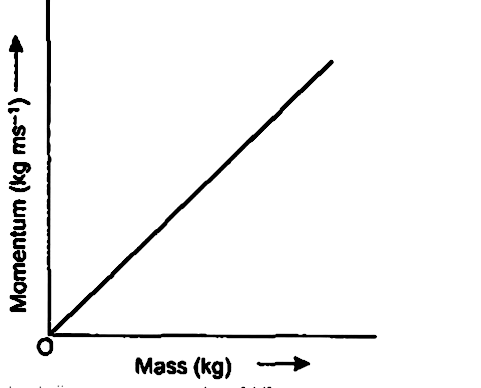Home/Class 9/Physics/

What is momentum? Write its $$SI$$ unit. Interpret force in terms of momentum. Represent the following graphically momentum versus mass when velocity is constant.Speed
00:00
05:32## QuestionPhysicsClass 9

What is momentum? Write its $$SI$$ unit. Interpret force in terms of momentum. Represent the following graphically momentum versus mass when velocity is constant.

Momentum of an object is the product of it's mass and it's velocity. It is a vector quantity.
Momentum$$= \text{mass} × \text{velocity}$$
i.e., $$p=\text{mv}$$
And the $$SI$$ unit of momentum is $$kg\ m\ s^{-1}$$
Force $$=$$ Rate of change in momentum
Graph for momentum vs mass when velocity is constant